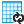You are viewing the RapidMiner Studio documentation for version 9.0 - Check here for latest version

#Pivot (RapidMiner Studio Core)

## Synopsis

This operator rotates an ExampleSet by grouping multiple examples of same groups to single examples.

## Description

The Pivot operator rotates the given ExampleSet by grouping multiple examples of same groups to single examples. The group attribute parameter specifies the grouping attribute (i.e. the attribute which identifies examples belonging to the groups). The resultant ExampleSet has n examples where n is the number of unique values of the group attribute. The index attribute parameter specifies the attribute whose values are used to identify the examples inside the groups. The values of this attribute are used to name the group attributes which are created during the pivoting. Typically the values of such an attribute capture subgroups or dates. The resultant ExampleSet has m regular attributes in addition to the group attribute where m is the number of unique values of the index attribute. If the given ExampleSet contains example weights (i.e. an attribute with weight role), these weights may be aggregated in each group to maintain the weightings among groups. This description can be easily understood by studying the attached Example Process.

## Differentiation

###Transpose

The Transpose operator simply rotates the given ExampleSet (i.e. interchanges rows and columns) but the Pivot operator provides additional options like grouping and handling weights.

## Input

•example set input (Data Table)

This input port expects an ExampleSet. It is the output of the Subprocess operator in the attached Example Process.

## Output

•example set output (Data Table)

The ExampleSet produced after pivoting is the output of this port.

•original (Data Table)

The ExampleSet that was given as input is passed without any modifications to the output through this port. This is usually used to reuse the same ExampleSet in further operators or to view the ExampleSet in the Results Workspace.

## Parameters

• group_attributeThis parameter specifies the grouping attribute (i.e. the attribute which identifies examples belonging to the groups). The resultant ExampleSet has n examples where n is the number of unique values of the group attribute. Range: string
• index_attributeThis parameter specifies the attribute whose values are used to identify the examples inside the groups. The values of this attribute are used to name the group attributes which are created during the pivoting. Typically the values of such an attribute capture subgroups or dates. The resultant ExampleSet has m regular attributes in addition to the group attribute where m is the number of unique values of the index attribute. Range: string
• consider_weightsThis parameter specifies whether attribute weights (if any) should be kept and aggregated or ignored. Range: boolean
• weight_aggregationThis parameter is only available when the consider weights parameter is set to true. It specifies how example weights should be aggregated in the groups. It has the following options: average, variance, standard_deviation, count, minimum, maximum, sum, mode, median, product. Range: selection
• skip_constant_attributesThis parameter specifies if the attributes should be skipped if their value never changes within a group. Range: boolean
• data_managementThis is an expert parameter. There are different options, users can choose any of them Range: selection

## Tutorial Processes

### Introduction to the Pivot operator

This Example Process starts with the Subprocess operator. There is a sequence of operators in this Subprocess operator that produces an ExampleSet that is easy to understand. A breakpoint is inserted after the Subprocess operator to show this ExampleSet. The Pivot operator is applied on this ExampleSet. The group attribute and index attribute parameters are set to 'group_attribute' and 'index_attribute' respectively. The consider weights parameter is set to true and the weight aggregation parameter is set to 'sum'. The group_attribute has 5 possible values therefore the pivoted ExampleSet has 5 examples i.e. one for each possible value of the group_attribute. The index_attribute has 5 possible values therefore the pivoted ExampleSet has 5 regular attributes (in addition to the group_attribute). Here is an explanation of values of the first example of the pivoted ExampleSet. The remaining examples also follow the same idea.

The value of the group_attribute of the first example of the pivoted ExampleSet is 'group0', therefore all values of this example will be derived from all examples of the input ExampleSet where the group_attribute had the value 'group0'. The ids of examples with 'group0' in the input ExampleSet are 12, 16, 19 and 20. In the coming explanation these examples will be called group0 examples for simplicity.

The value of the weight_attribute attribute of the pivoted ExampleSet is 11. It is the sum of weights of group0 examples i.e. 4 + 4 + 0 + 3 = 11. The weights were added because the weight aggregation parameter is set to 'sum'. The value of the value_attribute_index0 attribute of the pivoted ExampleSet is 4. Only two examples (id 12 and 16) of the group0 examples had 'index0' in index_attribute. The value of the latter of these examples (id 16) is selected i.e. 4 is selected. The value of the value_attribute_index1 attribute of the pivoted ExampleSet is 1. Only one example (id 19) of the group0 examples had 'index1' in index_attribute. Therefore its value (i.e. 1) is selected. The value of the value_attribute_index2 attribute of the pivoted ExampleSet is undefined because no example of the group0 examples had 'index2' in index_attribute. Therefore its value is missing in the pivoted ExampleSet. The value of the value_attribute_index3 attribute of the pivoted ExampleSet is 3. Only one example (id 20) of the group0 examples had 'index3' in index_attribute. Therefore its value (i.e. 3) is selected. The value of the value_attribute_index4 attribute of the pivoted ExampleSet is undefined because no example of the group0 examples had 'index4' in index_attribute. Therefore its value is missing in the pivoted ExampleSet.